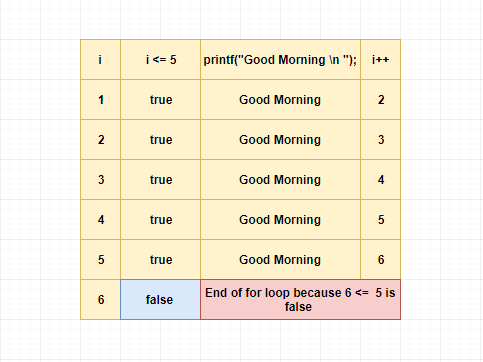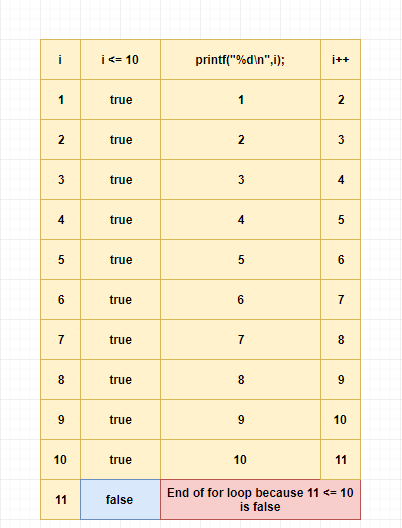# for loop in c

Loops are used in programming to repeat the execution of a specific block of code until the given condition becomes false.

In general, we should use for the loop when we know the exact loop count.

#### Example

1.Print “Good Morning” 10 times

Here we exactly know the loop iteration count which is 10.

2.Print numbers from 1 to 100

Here also we know the iteration count exactly which is 100.

## For Loop Syntax

```for (initialize; condition; increment or decrement)
{
//statements
}
```

## How for loop works?

#### 1. It will initialize the variable.

The initialization statement executed only once.

#### 2. It will check whether the given condition is true or not.

if the condition is true,

The statements under the for loop will be executed.

After that, the value of the variable will be incremented or decremented.

Again it will follow the above step(2) with the updated variable value.

else

The program execution comes out of the "for loop."

## For Loop Examples

#### Example 1

Printing “Good Morning” 5 times.

Example

```#include<stdio.h>

int main()
{
int i;

for(i=1;i<=5;i++)
{
printf("Good Morning\n");
}

return 0;
}
```

#### Pictorial Explanation#### Example 2

Print numbers from 1 to 10.

Example

```#include<stdio.h>

int main()
{
int i;

for(i=1;i<=10;i++)
{
printf("%d\n",i);
}

return 0;
}
```

#### Pictorial Explanation